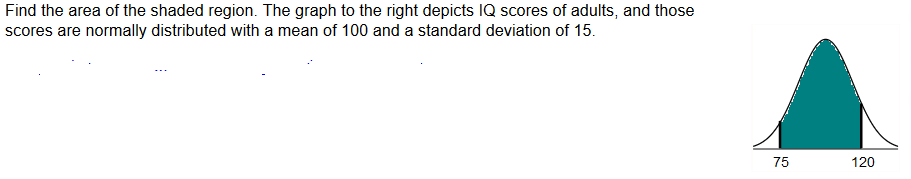# Find the area of the shaded region. The graph to the right depicts IQ scores of adults, and thosescores are normally distributed with a mean of 100 and a standard deviation of 1575120

Question
3213 viewshelp_outlineImage TranscriptioncloseFind the area of the shaded region. The graph to the right depicts IQ scores of adults, and those scores are normally distributed with a mean of 100 and a standard deviation of 15 75 120 fullscreen
check_circle

Step 1

The normal distribution:

A continuous random variable X is said to follow normal distribution if the probability density function of X is,

Step 2

Standard normal distribution:

The standard normal distribution is a special case of normal distribution. The standard normal distribution will have mean 0 and standard deviation 1. If a random variable y follows normal distribution with mean (m) and standard deviation (s), then the standard normal variable z will be as given below:

Step 3

Calculation:

From the given graph, it is seen that the shaded region is between the IQ scores 75 and 120.

Here, the IQ score, say X, is normally distributed with mean μ = 100 and standard deviation σ = 15. Thus, the p...

### Want to see the full answer?

See Solution

#### Want to see this answer and more?

Solutions are written by subject experts who are available 24/7. Questions are typically answered within 1 hour.*

See Solution
*Response times may vary by subject and question.
Tagged in
MathStatistics

### Other